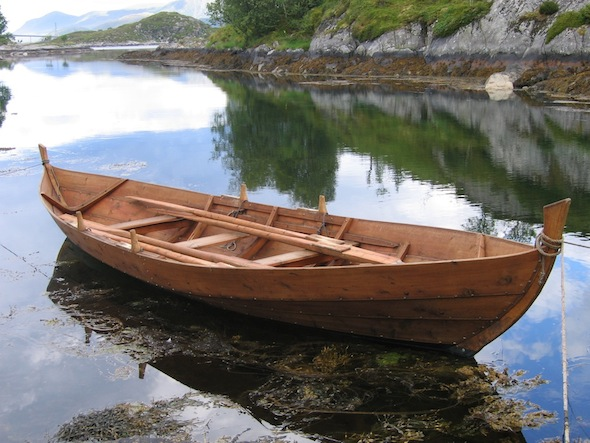# Row, Row, Row Your BoatYou and $n-1$ friends decide to take a trip to an island in a row boat. You'd like to get an idea of how long it will take you to get there.

Assume that you and each of your friends are perfectly identical, some kind of cookie cutter people, who can each provide a given level of power $P_p$ to row the boat. We'll suppose that the drag force is proportional to the submerged area of the boat, the square of the velocity, and the density of the water: $F_d\sim\rho A_{boat} v_{boat}^2$, i.e. skin drag. Finally, let's assume the boat is made of $n$ identical sections, of volume $V_p$, that each contribute equally to the surface area, i.e. $A_{boat}\sim \left(nV_p\right)^{2/3}$.

Under these assumptions, the steady state velocity of the boat can be written as $v_{boat} \sim n^{\alpha}P_p^{\beta}V_p^{\gamma}\rho^{\delta}$. The product of the exponents, $\alpha \beta \gamma \delta$, can be written as $a/b$ where $a$ and $b$ are two coprime integers, what is $a+b$?

Hint: Think of how energy is lost by the boat.

This is not an original

×